Open In App

# In a weighted graph, Is zero allowed as an edge’s weight?

In this article, we will discuss the overview of the Graph and will focus on a scenario wherein a weighted graph, Is zero allowed as an edge’s weight. Let’s discuss it one by one as follows.

Overview :
A graph is defined as a G(V, E) where V is a set of vertices and E is the set of edges. The interconnected point from one object to another object is called vertices, and a link that connects the vertices from one object to another is called edges.

Example –
Take a look at the following graph as follows.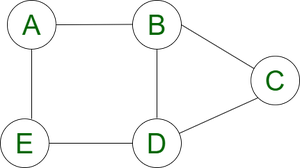Pictorial representation of a graph.

In the above graph G(V, E) as follows.

```Vertices(V)  = {A,B,C,D,E}
Edges(E)     = {AB,AE,ED,BD,BC,CD}```

Weighted Graphs :
A weighted graph is a graph such that each edge is labeled with a number, called the weight of that edge.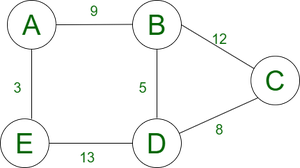Pictorial representation of a weighted graph

Example –
For example, the vertices of the graph above may represent certain towns in India, and the edges may represent roads between the towns, with their separation distances marked.

In a weighted graph, Is zero allowed as an edge’s weight :
The simple answer to the above question is that, If zero-weighted edges are helpful to you, you are free to use them but make sure you let the readers know about it. Below two cases represent that zero-weighted edge can or cannot use depends on the situation.

CASE-1: Zero weighted-edge is allowed –

Step-1 :
Suppose, in your graph, vertices represent a person. The edge-weight represents the money borrowed by one from another.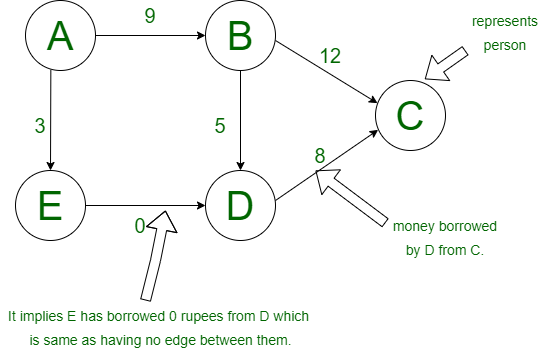Figure 1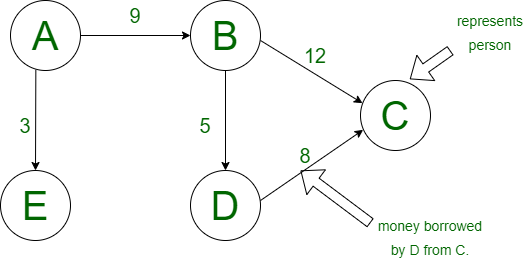Figure 2

Step-2 :
Then, in this case, the zero-weighted edge will represent that person-1 has borrowed money 0 from person-2, which would mean that person-1 has not borrowed any money from person-2, which is the same as having no edge.

Step-3 :
So, From the above figures, we can see that figure -1 and figure-2 are the same. In this case, the zero-weight edge is allowed.

CASE-2 :
Zero weighted-edge is not allowed –

Step-1 :
Suppose in your graph, vertices represent cities, and the edge represents the road between those cities, and the edge-weight represents the distance between the two cities.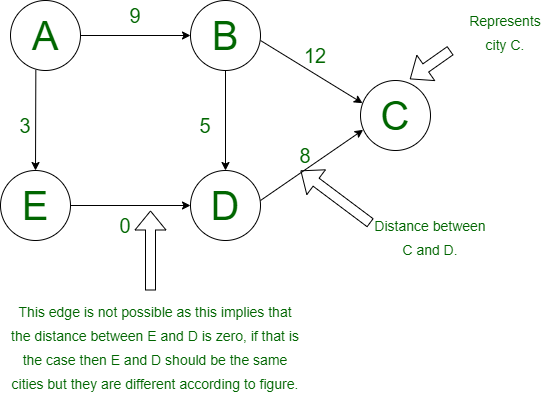Figure -3

Step-2 :
In that case, zero weighted-edge is not allowed, as the distance between cities E and D are zero so E and D should be the same cities that implies E should be equal to D, but that’s not possible, they are different so here we cannot use zero-weighted edge.

Step-3 :
We cannot use a zero-weighted edge here.